Pages: 4Words: 890

## Subject Code: BUMAN201A

Subject Name:Business Maths and Statistics Assessment:Project based Assessment

Course Name:Bachelor of Business, Bachelor & Diploma of Applied Commerce

This project will require students to apply statistical analysis and calculations to data and analyse the results of these calculations in a business setting.

Students must also submit an excel file with their calculations. This file must have formulas in the appropriate cells, and not data entered solutions. No marks will be awarded for written calculations.

Students are to include all parts of the question in their final report. Submit the Excel file in the Excel file upload section, submit the report in the report upload section. The report will be checked in Turnitin for plagiarism, please do not copy someone else’s work nor give your work to others. If plagiarism is detected both parties will be held accountable and appropriate marks will be deducted.

The assignment covers weeks 1 through to 10.

Structure of the assessment

This project will require students to apply statistical analysis and calculations to data and analyse the results of these calculations in a business setting. This assignment is to be presented as a report of no more than 8 pages A4 pages plus the appendices. The report should include all references and resources used in its compilation. All calculations for task 1, 2, 3 and 4 must be performed and presented using MS Excel and Word. The report shall include four sections to represent the four different tasks.

### Using the Excel data - ‘Sales’ sheet

1. Generate one table and one graph that provides a break up of all Sales Revenue by Region and Representative (in rows) and for all products (in the columns). The data presented in the table for each product/representative should be shown in dollars. A pivot table/chart is a great tool in Excel to do this. Make sure your graphs and tables look professional.
2. Generate one table that provides a break up of all Sales Revenue by Region and Representative (in rows) and for all products (in the columns). The data presented in the table for each product/representative should be shown in percentages of the grand total of sales revenue. Percentages should be to two decimal places.
3. Generate separate table for each of the 3 product categories. You will have table for Cricket Balls, a table for Stumps, and a table for Cricket Bats. Each table should include data for each representative within each region, detailing sales in units and revenue in dollars. Make sure your tables look professional.
4. Generate 3 pie charts, one for each of the product categories, detailing the sales for each region as a percentage of the total sales for each product category.
5. Generate 3 pie charts, one for each of the three regions detailing the sales for each representative as a percentage of the total region’s sales.
6. Comment on the data you have created. As the manager of this business, how could you use this data?

### Using the Excel data - ‘Weight of Tub’ sheet

You are the production manager for Yummy Yoghurt. Your production line produces 750g tubs of yoghurt for sale at supermarkets all over Australia.

Your company has a target that at least 95% of its yoghurt tubs will contain between 745g and 755g grams of yoghurt. Assume the distribution of the weight of yoghurt is normal.

1. Calculate the average and standard deviation of the tubs that have been measured. You should use excel formulas for this.
2. What is the probability that a tub will weigh between 745 and 755 grams?
3. What is the probability that a tub will weigh less than 740 or more than 760 grams?
4. What is the probability of a tub weighing less than 735 grams?
5. What is the probability of a tub weight over 765g grams?
6. Has the company meet their target? What are the consequences of not meeting this target? How can you use this data in your business? (approx. 250 words)

### Use the Excel Data ‘Hours of Attendance”

Student Services wants to undertake a full analysis of the relationship between class attendance hours during the semester and the Accounting final exam mark.

1. Undertake a full correlation analysis of the relationship between between class attendance hours during the semester and the Accounting final exam mark
2. Develop the linear regression model of the relationship between the between class attendance hours during the semester and the Accounting final exam mark. Include in the report a full detailed analysis concerning the model that has been fitted to the data.
3. Predict the expected Accounting final exam mark of a student who has attended 44 hours of the 48 scheduled hours of classes this semester.
4. Predict the Accounting final exam mark if a student attended 40 hours.
5. Comment on the reliability of this predicted final exam mark (approx. 50 words)
6. Suggest 3 other factors that may influence the Accounting final exam mark. (approx. 100 words)

Using the Census Data and Census Tables available on the Australian Bureau of Statistics  abs.gov.au , select a Census Data, Quick Stats and the suburb of your choice (notify teacher as soon as possible of your choice to ensure no duplications).

1. Copy and paste the Frequency Tables for the following variables and Construct appropriate graphs for each of them.
• Marital Status
• Gender
• Age
• Employment – hours worked
• Highest Educational attainment
• Languages spoken other than English
2. Using the Frequency Table for Grouped Age, locate the Age groups where Q1, Median and Q3 are located. Highlight the appropriate groups in the data table for each. Give an estimate of each of Q1, Median and Q3 and explain what each of them mean in relation to the Age of the sample.
3. What shape is the data for Age?
4. The data from the Australian Census of 2016 is now available from the Australian Bureau of Statistics. Determine 6 statistical snapshot facts that indicate some of major demographic changes in Australia in recent years. (approx. 400 words)

BUMAN201A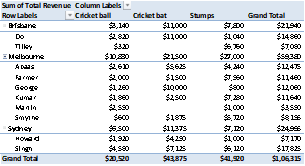Above pivot table and chart presents breakup of revenue for regions. Further, it is broken up by representatives and products also.Above pivot table and chart presents breakup of revenue for regions. Further, it is broken up by representatives and products also. The difference from previous section is that numbers are presented as percentage of total revenue.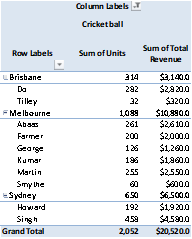The above pivot tables present information the units sold and revenue is presented for various regions, categorized by representatives. Filter on column labels has been used to create separate tables for separate products.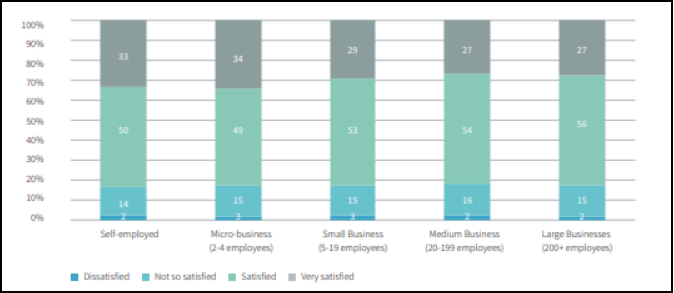The above pie charts present region-wise percentage of revenue for each of the three products.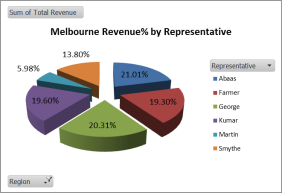The above pie charts present representative-wise percentage of revenue for each of the three regions.

The above data is useful in determining various factors, such as, what product is selling the maximum, which product is generating the maximum revenue, which region has maximum demand and of which product, which representative is performing well, etc. Such information can be further utilized for forecasting purposes, appraisal of representatives, marketing strategy etc.

Task 2: Weight of Tub Sheet

For calculating various probabilities, z score has been calculated using formula (x-Mean/SD). Since it is a normal distribution, z table has been used to find corresponding probabilities as follows:Hence,

1. Using given data, the average is 750.75gms while standard deviation is 7.17gms.
2. There is 51.2% probability that tub weight will be between 745gms and 755gms.
3. There is 16.5% probability that tub weight will be less than 740gms or more than 760gms.
4. There is 1.4% probability that tub weight will be less than 735gms
5. There is 2.3% probability that tub weight will be more than 765gms.
6. As seen above, there is only 51.2% probability that the tub weight is between 745gms and 755gms. Hence, the company is not meeting its target that at least 95% of the tubs will be in this range. The consequence is the variation in tubs as seen in high standard deviation. This may cause dissatisfaction in customers as they will receive different quantity despite paying same price. Additionally, the company quality will be under question due to so much of variation. The company must make efforts to streamline the process and achieve more precision in filling the tubs.

1. The correlation tool in excel was used :It can be seen that the correlation between two variables, namely, hours of attendance and marks, is high and positive at 0.6534. This indicates that a unit increase in hours of attendance will lead to 0.65 unit increase in marks. Also, a unit decrease in hours of attendance will lead to 0.65 unit decrease in marks.

(b) The regression tool in excel was used:The above indicates same value for R as correlation, that is, 0.653.

The regression equation is: y= 15.13 + 1.37 x1

where,

• Dependent variable, Marks is represented as: y
• Independent variable, Hrs of Attendance is represented as x1

The above equation has a constant of 15.13 which is also known as Y-intercept coefficient. It is the minimum value for y even when all x values are zero. It is the point where regression line crosses the vertical axis and is also known as ‘β0’ or ‘constant’. Also, x1 is greater than zero indicating that the relationship is positive such that x and y increase or decrease together.

The output also provides coefficients and other statistically significant information, such as p-values for each of the coefficients. These values help in determining whether the variable has statistically significant relationship with the dependent variable or not.

Each of the p-value entails a null hypothesis that the variable has no correlation with the dependent variable. The alternative hypothesis is that the variable has correlation with the dependent variable. In above, the p-value for both intercept (0.0013) and x variable (0.0000) is less than assumed significance level of 0.05, indicating that there is correlation between the two variables.

(c) The regression equation is: y= 15.13 + 1.37 x1

Now, if x = 44, y= 15.13 + 1.37*44 = 75.29.

Hence, a student who attended 44 hours can be predicted to get 75.29 marks.

(d) The regression equation is: y= 15.13 + 1.37 x1

Now, if x = 40, y= 15.13 + 1.37*40 = 69.82.

Hence, a student who attended 40 hours can be predicted to get 69.82 marks.

(e) From the above output, the ANOVA output helps to understand reliability of the regression model generated. The F value is 132.62 while Significance F is 0.000. Hence, it can be concluded that regression model is reliable. In other words, the above predictions through the regression model are also reliable.

(f) Some of the other factors that can impact marks are: number of hours studied, difficulty level of questions in the exam, and number of hours of sleep before the exam.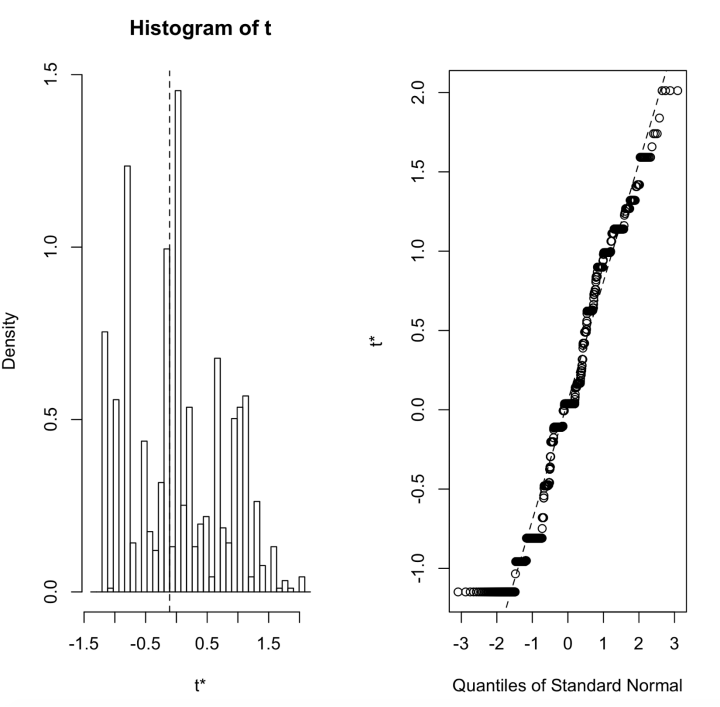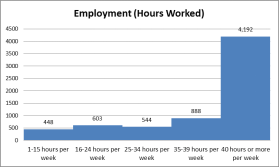The selected region is Bondi Beach, NSW (State Suburb). The required graphic representations are:

1. The age group table is as follows:
 Age f Cf 0-4 years 591 591 5-9 years 411 1002 10-14 years 285 1287 15-19 years 240 1527 20-24 years 735 2262 25-29 years 1,952 4,214 30-34 years 2,162 6,376 35-39 years 1,336 7,712 40-44 years 964 8676 45-49 years 684 9360 50-54 years 516 9876 55-59 years 437 10313 60-64 years 330 10643 65-69 years 325 10968 70-74 years 208 11176 75-79 years 169 11345 80-84 years 136 11481 85 years and over 178 11659 11659

Median = 11659/2 = 5829.5. This will lie in group 30-34 years.

Q1 step 1 = 11659/4 = 2914.75 = This will lie in group 25-29 years.

Q1 step 2 = 25 + ((2914.75-2262)/1952)*4

Q1 = 26.3 years

Q3 step 1 = 3*(11659/4) = 8744.25 = This will lie in group 45-49 years.

Q3 step 2 = 45 + ((8744.25-8676)/684)*4

Q3 = 45.4 years

(c) As can be seen, the data for age is normally distributed:Tags: